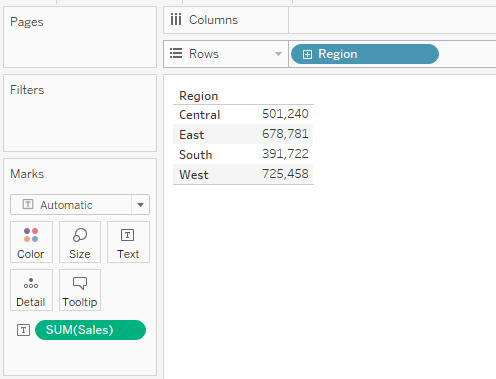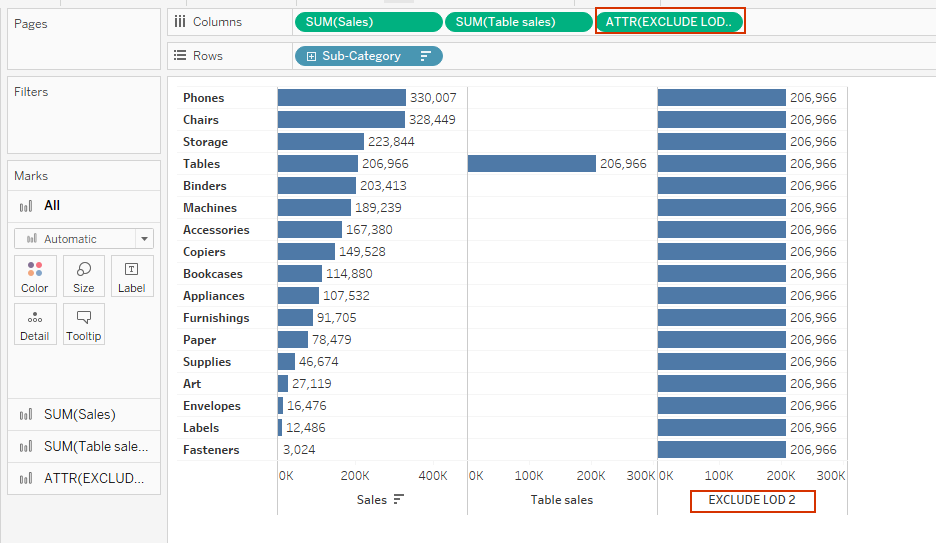Search
• Bernard

# How to use EXCLUDE level of detail (LOD) expression in TableauLevel of detail (LOD) expressions allows Tableau users to compute values both at data source level and visualization level giving users more control on the level of granularity they can compute data.

EXCLUDE LOD expression, which is one of the Tableau LODs is executed by removing some of the detail from the view when computing the values. In other words, the EXCLUDE LOD expression prevents the calculation from using certain dimensions present in the view.

In the following two examples – I’ll share different ways you can use the EXCLUDE LOD expressions.

### Example 1: Computing percent of total

Using the Sample-Superstore data set, drag Region to the rows and Sales to the text.Next, I’ll need to compute what will be the denominator of my calculation (the sales for all regions) using the following calculation.(Note, the above calculation excludes the dimension Region when computing the sales)

Adding the above calculation to the view returns the same figure across all regions (that is the totals sales for all regions)And now I can use the EXCLUDE LOD expressions to compute the percent of total as shown below.Adding this calculation to the view and formatting it as a percent – gives us the proportion each region contributed to the total sales.### Example 2: Computing difference

Let’s say using Sample-Superstore data you’ve created the view below - showing sales by sub-category.Let’s say, with this view – you want to compute the difference in sales between the Sub-category Table and other Sub-categories. You can achieve that using the EXCLUDE LOD.

To do that, lets compute the sales for sub-category Table using the formula below.Adding the above calculation to the view returns the sales for Table sub-category.Next, using the EXCLUDE LOD – lets exclude the dimension sub-category while computing the sales for Table sub-category. This calculation will replicate the Table sales across all other Sub-categories.

See the formula below.Adding this calculation to the view.And now, we can compute the difference using the formula below.Adding this calculation to the view - we’ve the difference in sales between Tables and other product sub-categories.